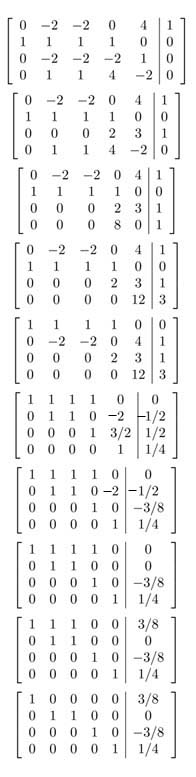SEARCH HOMEMath Central Quandaries & QueriesMuhammad, a student: -2B-2C+4E=1 A+B+C+D=0 -2B-2C-2D+E=0 B+C+4D-2E=0I've put your question in matrix form (first column is A, second column is B, third column is C, fourth column is D, fifth column is E and the last is the constant term). I find it a little easier to write a question like this as an augmented matrix.Here is your question in matrix form I subtracted the first row to the thrid row, put the result in the thrid row I multiplied the last row by 2 then added it to the first row, put the result in the last row I multiplied the thrid row by -4 then added it to the last row, put the result in the last row I switched the first and second row. I divided the second row by -2, the thrid row by 2 and the last row by 4 I multiplied the last row by -3/2 and then added it to the thrid row, I put the result in the thrid row I multiplied the last row by 2 then added it to the second row, I put the result in the second row I subtracted the second row from the first row and put the result in the first row Now the matrix is in row echelon form. We can see that A=3/8, B=-C, D=-3/8 and E =1/4. Note that B can be any number and C is the negative of B.

Check out "An Augmented Matrix" for further help

Hope this helps,

JaniceMath Central is supported by the University of Regina and The Pacific Institute for the Mathematical Sciences.